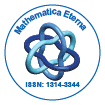# Mathematica EternaOpen Access

ISSN: 1314-3344

## Abstract

### A Class of Univalent Harmonic Mappings

Jinjing Qiao and Qiannan Guo

The main aim of this paper is to investigate a class of univalent harmonic mappings, which is the generalization of the class of analytic functions whose derivative has a positive real part. The distortion theorem, the radius of convexity, univalence of the partial sums, the extremal property of f0(z) = −z − 2 log(1 − z), and functions with initial zero coefficients are considered.

Top